TitleIntermediate Algebra
Answer/Discussion to Practice Problems
Tutorial 11: Practice Test on Tutorials 7 - 10

 Problems 1a - 1c:  Solve for the variable.

 1a.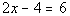Answer: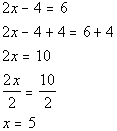1b.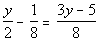Answer: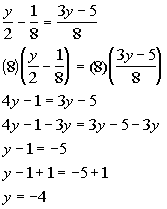1c.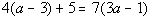Answer: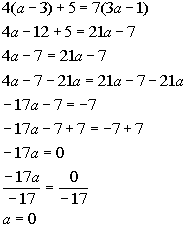Be careful on this one.  Remember that you can divide into 0.  0 divided by anything is 0.  So the answer is a = 0, NOT no solution.  If you were trying to divide by 0, for example -17/0, then the answer would be no solution.

 Problems 2a - 2c:  Solve the word problem.

 2a.  The product of 5 and a number is 8 less than 7 times that numberAnswer:

 Step 1: Understand Make sure that you read the question carefully several times.  Since we are looking for a number, we will let  x = a number

 Step 2: Devise a Plan (Translate)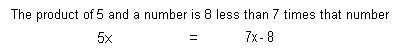Step 3: Carry out the plan (solve)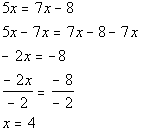Step 4: Look back (check and interpret) If you take 5 times 4, it is 8 less than 7 times 4.  So, this does check.   FINAL ANSWER:  The number is 4.

 2b.  The sum of 3 consecutive integers is 234.  Find the integers.Answer:

 Step 1: Understand Make sure that you read the question carefully several times.  We are looking for 3 consecutive integers, we will let x = 1st consecutive integer x + 1 = 2nd consecutive integer x + 2  = 3rd  consecutive integer

 Step 2: Devise a Plan (Translate)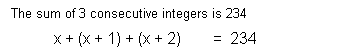Step 3: Carry out the plan (solve)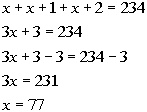Step 4: Look back (check and interpret) If you take the sum of 77, 78 and 79, you do get 234.   FINAL ANSWER:  The three consecutive integers are 77, 78 and 79.

 2c.   You are wanting to buy a stereo system that costs \$325 plus tax.  If you are going to be taxed 8.25%, how much will be the final cost of the stereo system? (round to the nearest cent)Answer:

 Step 1: Understand Make sure that you read the question carefully several times.  Since we are looking for the final cost of the stereo system, let x = the final cost

 Step 2: Devise a Plan (Translate)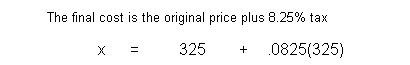Step 3: Carry out the plan (solve)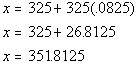Step 4: Look back (check and interpret) If you add on 8.25% of 325 to 325 you do get 351.8125.   FINAL ANSWER:  The final cost is \$351.81.

 Problem 3a:  Solve the equation for the  specified variable.

 3a.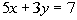for yAnswer: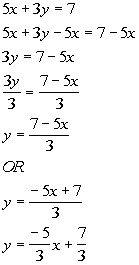Problems 4a - 4b:  Solve the following word problems.

 4a  You are traveling down I-40 at a constant speed of 70 mph.  How many hours will it take you to travel 385 miles?Answer:

 Step 1: Understand Make sure that you read the question carefully several times.  Since we are looking for how long it would take you to travel, we can use the distance/rate formula: d = rt The variables in this formula represent the following: d = distance r = rate t = time

 Step 2: Devise a Plan (translate) In this problem,  d = 385 r = 70 t =  ? = this is the variable we are looking for d = rt Plugging the values into the formula we get: 385 = 70t

 Step 3: Carry out the plan (solve)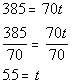Step 4: Look back (check and interpret) If you go a rate of 70 miles per hour for 5.5 hours, you would travel 385 miles.   FINAL ANSWER:  It would take 5.5 hours.

 4b.  A farmer has three cylindrical shaped containers to hold feed in.  Each container has the same radius of 3 feet and height of 4 feet.  What is the total volume of the three containers? Hint: the volume formula for a cylinder is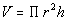Answer:

 Step 1: Understand Make sure that you read the question carefully, several times.  Since we are needing to find the volume of a cylinder,  we can use this formula:The variables in this formula represent the following: V = volume of a cylinder r = radius h = height

 Step 2: Devise a Plan (translate) In this problem,  V = ? = this is the variable we are looking for r = 3  h = 4Plugging the values into the formula we get: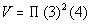Step 3: Carry out the plan (solve) First, find the volume of a cylinder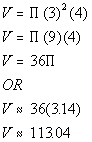Each cylinder has a volume of 36cubic feet  or approximately 113.04 cubic feet.  If there are three of them, then we need to multiply this volume by 3.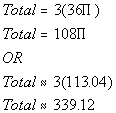Step 4: Look back (check and interpret) If you take 4times 3 squared, you do get 36, so the volume checks out.  If you take 3 times 36, you do get 108, so the volume for three cylinders checks out.   FINAL ANSWER:  The total volume for three cylinders is 108cubic feet  or approximately 339.12 cubic feet.

 Problems 5a - 5c :  Solve, write your answer in interval notation and graph the solution set.

 5a.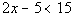Answer: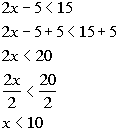Interval notation:  (-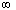, 10) Graph: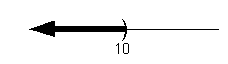5b.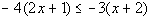Answer: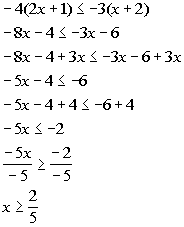Interval notation: [2/5,) Graph: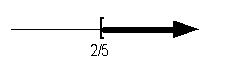5c.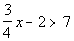Answer: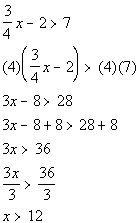Interval notation:  (12,) Graph: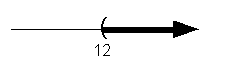Last revised on July 3, 2011 by Kim Seward.
All contents copyright (C) 2001 - 2011, WTAMU and Kim Seward. All rights reserved.